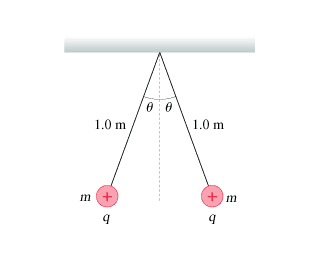# MasteringPhysics: Find the angle between the point charges.

## Homework StatementTwo m = 4.0 g point charges on 1.0-m-long threads repel each other after being charged to q = 80 nC , as shown in the figure. What is the angle θ? You can assume that θ is a small angle.

Fe=kq2/r2
F=ma
Fg=mg

## The Attempt at a Solution

Equation 1:[/B]
r = 2sinθ
Fe=kq2/(2sinθ)2
Equation 2:
tanθ=Fe/Fg
Fgtanθ=Fe
Combining Them:
Fgtanθ=kq2/(2sinθ)2
Fgtanθ=kq2/(4(sinθ)2)
(sinθ)2tanθ=kq2/(4Fg)
(sinθ)2tanθ=kq2/(4mg)

Okay, so now I have an equation with all the thetas on one side. So I tried to plug it into Wolfram Alpha to solve for theta, but it is giving me some really weird results. I'm not quite sure where I went wrong. Any help is greatly appreciated! Here's the Wolfram Alpha link. Thanks!

http://www.wolframalpha.com/input/?...*(8*10^-9)^2/(4*4*10^-3*9.8)+for+x+in+degrees

cnh1995
Homework Helper
Gold Member
I didn't check your equations, but
You can assume that θ is a small angle.
..this means you can use,

I didn't check your equations, but

..this means you can use,
Hm, I tried that and it didn't seem to work unfortunately.
Why is it that I can make that assumption anyway?

EDIT: Just used all my attempts and got that the correct answer is 4.1 degrees. Still need to figure this out since it's for studying.

cnh1995
Homework Helper
Gold Member
Hm, I tried that and it didn't seem to work unfortunately.
Why is it that I can make that assumption anyway?
It comes from the Maclaurin series expansion for sinθ and cosθ, where θ is in radian.
sinθ=θ-(θ3/3!)+(θ5/5!)-..

cosθ=1+(θ2/2!)+θ4/4!+...

You can see for small angle θ, sinθ≈θ and cosθ≈1 as higher order terms can be safely neglected.
Hence, tanθ≈θ.

it didn't seem to work unfortunately.

vela
Staff Emeritus
Homework Helper
Your work is fine. You should get the correct answer if you use cnh1995's suggestion. (I did.)

Your work is fine. You should get the correct answer if you use cnh1995's suggestion. (I did.)
Hmmm. 4.1 degrees? Can you look at my link here and see what I entered wrong?

Thank you all for helping me through this btw!

vela
Staff Emeritus